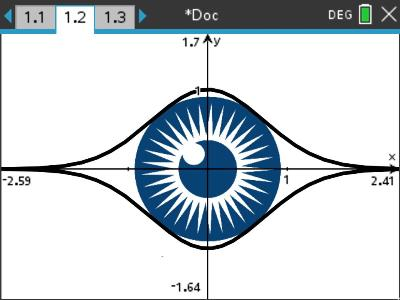# Activities

•• ##### AuthorAust Senior

50 Minutes

• ##### Device
•TI-Nspire™ CX CAS
•TI-Nspire™ CX

4.5

## Eye Spy#### Activity Overview

Students use calculus to determine the maximum size of the iris (circle) that just fits inside an outline of the eye defined by two bell shaped curves. The activity uses some basic differential calculus, introduces simple substitutions to eliminate variables and handy techniques for simplifying problems. The problem is much easier than it looks!

#### Objectives

Use calculus to determine the maximum area of a circle that just fits inside another curve. The combined curves appear as an eye adding some visual interest to the problem. The activity also introduces some techniques that students may use to help solve other, more complicated problems.

#### Vocabulary

• Differential Calculus
• Exponential function